# FAQs: THINK Points in Imagine Math 3+

As Imagine Math 3+ students work through lessons, they earn THINK Points they can redeem in the program in various ways. Below are some Frequently Asked Questions (FAQs) about THINK Points.

## 1. How do students earn points?

Students earn 250 THINK Points for taking the Imagine Math Benchmark, and various points for answering questions while working through their lesson. The points are applied to their total number of points after they have completed the entire lesson, which motivates students to finish the full lesson before spending their points.

Note

At this time, students do not earn THINK Points for taking the NWEA Map Growth Assessment.

Students also earn bonus THINK Points for testing out of lessons by scoring 80% or higher on the Pre-Quiz and for completing lessons:

• Test out of the lesson on the first attempt at the lesson - 750 bonus points
• Test out on the second attempt at the lesson - 500 bonus points
• Test out on the third attempt at the lesson - 250 bonus points
• Complete a lesson - 250 bonus points (for passed lessons only)

## 2. How many THINK Points do students earn for each answer?

To see the point breakdown for each lesson component, click on its name in the table below.

Pre-Quiz and Post-Quiz
During the Pre-Quiz and Post-Quiz, points are awarded after students answer each question as follows:
• Correct answer, first attempt at lesson - 25 points
• Correct answer, second attempt at lesson - 13 points
• Correct answer, third attempt at lesson - 7 points

No points are given for incorrect answers on Pre-Quizzes and Post-Quizzes, and students may not attempt the question again if they get it wrong the first time.

Note

The bonus points for testing out of lessons are awarded in addition to the points for each correct answer on the Pre-Quiz. In the example below, Missy answered 8 questions correctly on the first attempt at the Pre-Quiz and earned 25 points for each one, for a total of 200 points on the Pre-Quiz. The 750 bonus points for testing out of the lesson on the first try show as a separate line item in her THINK Point Ledger.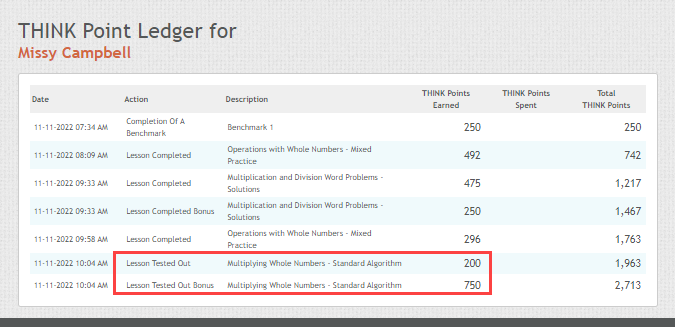Warm Up Game
During the Warm Up game, students can earn up to 75 points based upon questions they answer correctly within the allotted time frame. They earn 0 points if they choose to skip the Warm Up game.
Guided Learning and Practice
In the Guided Learning and Practice portions of each lesson, more points are awarded for correct answers on the first try of a lesson, and students receive fewer points for correct answers when they are retaking a lesson. Students must attempt the questions in these portions of the lessons multiple times if they don't get it right the first time.

First lesson attempt:

• Correct answer, first try - 25 points
• Correct answer, second try - 10 points
• Correct answer, third try - 5 points
• Correct answer on fourth try, or incorrect answer - 1 point

Second time taking the lesson (Retake)

THINK Points awarded for answers on the second attempt of a lesson are roughly half of what is awarded on the first attempt.

• Correct answer, first try - 13 points
• Correct answer, second try - 5 points
• Correct answer, third try - 3 points
• Correct answer on fourth try, or incorrect answer - 1 point

Third time taking the lesson (Deferred to end of student's pathway)

THINK Points awarded for answers on the third attempt of a lesson are roughly one fourth of what is awarded on the first attempt.

• Correct answer, first try - 7 points
• Correct answer, second try - 3 points
• Correct answer, third try - 2 points
• Correct answer on fourth try, or incorrect answer - 1 point

## 3. How are THINK Points from contests awarded?

In addition to the bonus THINK Points mentioned in question 1, students can earn bonus THINK Points by participating in contests such as Friday Night Math Nights. The contests and eligibility criteria are listed in the Contests section of the Imagine Math dashboard for teachers and administrators. Students can explore current contests in the Contests tab of their Imagine Math account.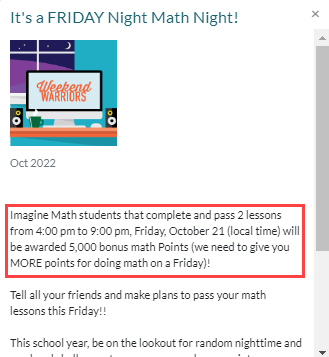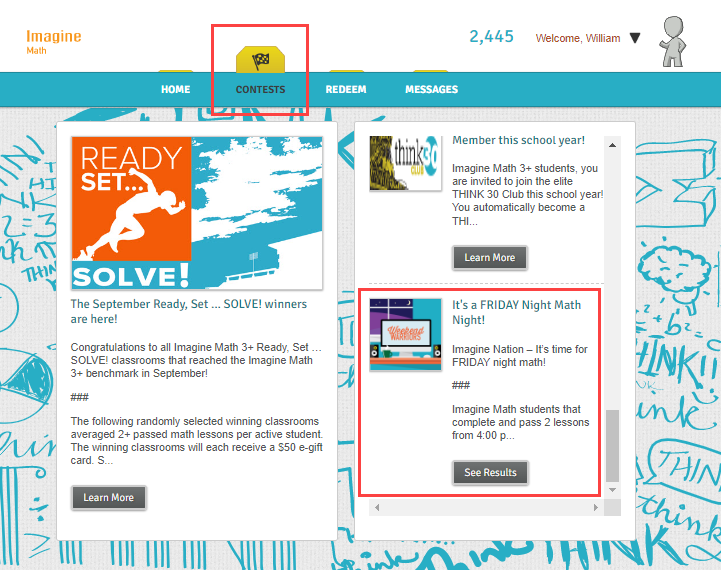Bonus THINK Points for contests are automatically added to the student's account at midnight of the qualifying day.

Caution

Bonus THINK Points do not reflect on any teacher or administrator reports because of the way they are allocated in Imagine Math. Only points earned in pathway lessons are reflected in the reports. However, students can view their bonus points in their THINK Point Ledger

## 4. How can students spend their THINK Points?

Students can choose to spend their THINK Points on designing their avatars, donating to the classroom goal, or giving to charity

## 5. How many THINK Points equal a dollar in charity donations?

The amount of THINK Points that equal a dollar varies according to the time of year and the specific charity. To view the points-to-dollar conversion rate for each monthly charity, view the Contests portion of the Imagine Math dashboard.

## 6. Where do students see their THINK Points?

Students can view their own points on the Home tab and in the THINK Point Ledger. They can also see Point Leaders on the student leaderboard

When students begin a new lesson, the point counter above their name reverts to 0, even when students have points from other lessons. This is because the number of THINK Points that displays at the top of the screen is the number of points they have earned so far in that lesson only. The number will increase as students work through the lesson.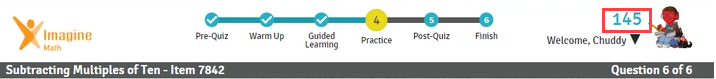When students finish the lesson and return to their Home tab, the number of Think Points reverts back to the total number of spendable points the student has.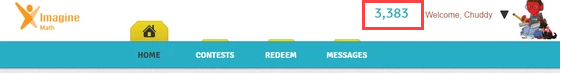## 7. Where do educators see students' THINK Points?

Educators can view students' THINK Points by customizing the Overview report. They can also view Point Leaders on the leaderboard.

## 8. Does changing a student's pathway affect their THINK Points?

No. Adding, removing, or switching a pathway in no way affects the THINK Points a student has already earned.